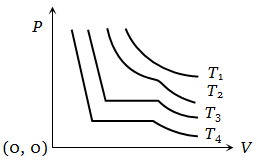NEET Physics Kinetic Theory of Gases Questions Solved

The change in volume V with respect to an increase in pressure P has been shown in the figure for a non-ideal gas at four different temperatures  and ${\mathrm{T}}_{4}$ . The critical temperature of the gas is1. ${\mathrm{T}}_{1}$

2. ${\mathrm{T}}_{2}$

3. ${\mathrm{T}}_{3}$

4. ${\mathrm{T}}_{4}$

Explanation is a part of a Paid Course. To view Explanation Please buy the course.

Difficulty Level: